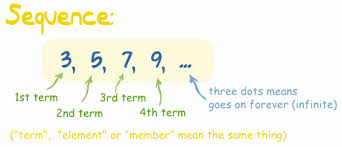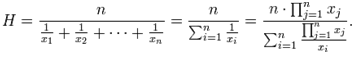×#### Thank you for registering.

One of our academic counsellors will contact you within 1 working day.

Click to Chat

1800-1023-196

+91-120-4616500

CART 0

• 0

MY CART (5)

Use Coupon: CART20 and get 20% off on all online Study Material

ITEM
DETAILS
MRP
DISCOUNT
FINAL PRICE
Total Price: Rs.

There are no items in this cart.
Continue Shopping• Complete JEE Main/Advanced Course and Test Series
• OFFERED PRICE: Rs. 15,900
• View Details

```Revision Notes on Sequence and Series

A sequence is a function of natural numbers with codomain as the set of real numbers or complex numbers.

A sequence may be finite or infinite depending on whether it has finite or infinite terms.A progression is a sequence having its terms in a definite pattern.

A Series is constructed by adding or subtracting the terms of a sequence.

A series having finite number of terms is called a finite series while the series having infinite number of terms is called infinite sereis.

Arithmetic progression and Arithmetic Mean

If ‘a’ is the first term and ‘d’ is the common difference of the arithmetic progression then its nth term is given by an = a + (n-1)d

In an = a + (n-1)d, note that:

if d > 0, then it is an increasing A.P.

if d < 0, then it is a decreasing A.P.

if d = 0, then all the terms remain same.

The sum, Sn of the first ‘n’ terms of the A.P. is given by Sn = n/2 [2a + (n-1)d]

If Sn is the sum of n terms of an A.P. whose first term is ‘a’ and last term is ‘l’,Sn = (n/2)(a + l).

If common difference is d, number of terms n and the last term l, then Sn = (n/2)[2l-(n -1)d]

If a fixed number is added or subtracted from each term of an A.P., then the resulting sequence is also an A.P. and it has the same common difference as that of the original A.P.

If each term of A.P is multiplied by some constant or divided by a non-zero fixed constant, the resulting sequence is an A.P. again.

The sum of two terms of an A.P. equidistant from the beginning and end is constant and equal to the sum of first and last terms.

If a1, a2, a3, …, an and b1, b2, b3, …, bn, are in A.P. then a1+b1, a2+b2, a3+b3, ……, an+bn and a1–b1, a2–b2, a3–b3, ……, an–bn will also be in A.P.

Suppose a1, a2, a3, ……,an are in A.P. then an, an–1, ……, a3, a2, a1 will also be in A.P.

If nth term of a series is tn = An + B, then the series is in A.P.

If a1, a2, a3, ……, an are in A.P., then a1 + an = a2 + an–1 = a3 + an–2 = …… and so on.

In order to assume three terms in A.P. whose sum is given, they should be assumed as a-d, a, a+d.

Four terms of the A.P. whose sum is given should be assumed as a-3d, a-d, a+d, a+3d

Five convenient numbers in A.P. a–2b, a–b, a, a+b, a+2b.

In general, we take a-rd, a-(r-1)d, …., a-d, a, a+rd in case we have to take (2r + 1) terms in an A.P.

Likewise, any 2r terms of an A.P. should be assumed as: a – (2r-1)d, a – (2r – 3)d, …., a – d, a, a + d, ………….. , a + (2r – 3)d, a + (2r – 1)d.

The arithmetic mean of two numbers ‘a’ and ‘b’ is (a+b)/2.

The terms A1, A2, ….. , An are said to be arithmetic means between a and b if a, A1, A2, ….. , An, b is an A.P.

Clearly, ‘a’ is the first term, ‘b’ is the (n+2)th term and ‘d’ is the common difference. Then, we have b = a+(n+2-1)d = a+(n+1)d

Hence, this gives ‘d’ = (b-a)/(n+1)

Geometric Progression and Geometric Mean

If ‘a’ is the first term and ‘r’ is the common ratio of the geometric progression, then its nth term is given by an = arn-1

The sum, Sn of the first ‘n’ terms of the G.P. is given by Sn = a (rn – 1)/ (r-1), when r ≠1; = na , if r =1

If -1 < x < 1, then lim xn = 0, as n →∞. Hence, the sum of an infinite G.P. is 1 + x + x2+ ….. = 1/(1-x)

If -1 < r< 1, then the sum of the infinite G.P. is a +ar+ ar2+ ….. = a/(1-r)

If each term of the G.P is multiplied or divided by a non-zero fixed constant, the resulting sequence is again a G.P.

If each term of the G.P is raised to the same power then the resulting sequence is again a G.P

If a1, a2, a3, …. and b1, b2, b3, … are two geometric progressions, then a1b1, a2b2, a3b3, …… is also a geometric progression and a1/b1, a2/b2, ... ... ..., an/bn will also be in G.P.

Suppose a1, a2, a3, ……,an are in G.P. then an, an–1, an–2, ……, a3, a2, a1 will also be in G.P.

Taking the inverse of a G.P. also results a G.P. Suppose a1, a2, a3, ……,an are in G.P then 1/a1, 1/a2, 1/a3 ……, 1/an will also be in G.P

If we need to assume three numbers in G.P. then they should be assumed as   a/b, a, ab  (here common ratio is b)

Four numbers in G.P. should be assumed as a/b3, a/b, ab, ab3 (here common ratio is b2)

Five numbers in G.P. a/b2, a/b, a, ab, ab2  (here common ratio is b)

If a1, a2, a3,… ,an is a G.P (ai > 0 ∀i), then log a1, log a2, log a3, ……, log an is an A.P. In this case, the converse of the statement also holds good.

If three terms are in G.P., then the middle term is called the geometric mean (G.M.) between the two. So if a, b, c are in G.P., then b = √ac is the geometric mean of a and c.

Likewise, if a1, a2, ……,an are non-zero positive numbers, then their G.M.(G) is given by G = (a1a2a3 …… an)1/n.

If G1, G2, ……Gn are n geometric means between and a and b then a, G1, G2, ……,Gn b will be a G.P.

Here b = arn+1 ⇒ r = n+1√b/a, Hence, G1 = a. n+1√b/a, G2 = a(n+1√b/a)2,…, Gn = a(n+1√b/a)n.

Relation between A.M. and G.M. is A.M. ≥ G.M.

Arithmetic Geometric Progression

A sequence of the form S = a + (a+d)r + (a+2d)r2 + (a+3d)r3 + …. is called an arithmetic geometric progression.

Here, each term is the product of corresponding terms in an arithmetic and geometric series.

Tn = [a + (n-1) d]rn-1 is the nth term of A.G.P.

Sum of n terms of A.G.P. is given by S = a/(1-r) + dr(1-rn-1/(1-r)2) – [a+(n-1)d]rn/(1-r), where r is the common ratio, d is the common difference and a is the first term.

S∞ = a/(1-r) + dr/(1-r)2.

Harmonic Progression and Harmonic Mean

Let a, b and c form an H.P. Then 1/a, 1/b and 1/c form an A.P.

If a, b and c are in H.P. then 2/b = 1/a + 1/c,  which can be simplified as b = 2ac/(a+c).

If ‘a’ and ‘b’ are two non-zero numbers then the sequence a, H, b is a H.P.

The n numbers H1, H2, ……,Hn are said to be harmonic means between a and b, if a, H1, H2 ……, Hn, b are in H.P. i.e. if 1/a, 1/H1, 1/H2, ..., 1/Hn, 1/b are in A.P. Let d be the common difference of the A.P., Then 1/b = 1/a + (n+1) d ⇒ d = a–b/(n+1)ab.

Thus 1/H1 = 1/a + a–b/(n+1)ab,

1/H2 = 1/a + 2(a–n)/(n+1)ab,

………..     ……….

1/Hn = 1/a + n(a–b)/(n+1)ab.

If x1, x2, … xn are n non-zero numbers, then the harmonic mean ‘H’ of these numbers is given by 1/H = 1/n (1/x1 + 1/ x2 + ……. +1/ xn)As the nth term of an A.P is given by an = a + (n-1)d, So the nth term of an H.P is given by 1/ [a + (n -1) d].

If we have a set of weights w1, w2, …. , wn associated with the set of values x1, x2, …. , xn, then the weighted harmonic mean is defined asQuestions on Harmonic Progression are generally solved by first converting them into those of Arithmetic Progression.

If ‘a’ and ‘b’ are two positive real numbers then A.M x H.M = G.M2

The relation between the three means is defined as A.M > G.M > H.M.

If we need to find three numbers in a H.P. then they should be assumed as 1/a–d, 1/a, 1/a+d

Four convenient numbers in H.P. are 1/a–3d, 1/a–d, 1/a+d, 1/a+3d

Five convenient numbers in H.P. are 1/a–2d, 1/a–d, 1/a, 1/a+d, 1/a+2d

Miscellaneous Sequences

Sequences containing Σn, Σn2, Σn3

?   Σn = n(n+1)/2

Σn2 = n(n+1)(2n+1)/6

Σn3 = [n(n+1)/2]2

Using method of difference:

If T1, T2,T3 are the terms of sequence then the terms T2 – T1, T3 – T2, T4 – T3 …..may at times be in A.P. or sometimes may be in G.P. In such series, we first compute their nth term and then compute the sum to n terms, using sigma notation.

A series in which each term is composed of the reciprocal of the product of r factors in A.P., the first factor of the several terms being in the same A.P., then such series are solved by splitting the nth term as a difference of two.

```### Course Features

• 728 Video Lectures
• Revision Notes
• Previous Year Papers
• Mind Map
• Study Planner
• NCERT Solutions
• Discussion Forum
• Test paper with Video Solution